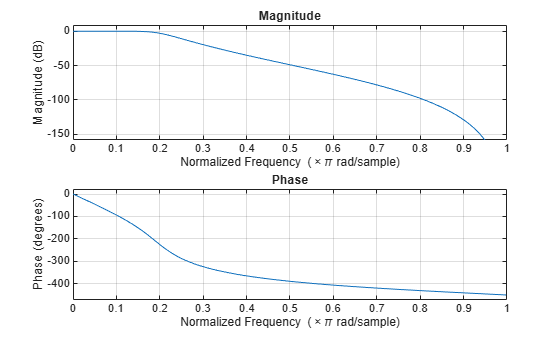# zp2sos

Convert zero-pole-gain filter parameters to second-order sections form

## Syntax

```[sos,g] = zp2sos(z,p,k) [sos,g] = zp2sos(z,p,k,'order') [sos,g] = zp2sos(z,p,k,'order','scale') [sos,g] = zp2sos(z,p,k,'order','scale',zeroflag) sos = zp2sos(...) ```

## Description

`zp2sos` converts a discrete-time zero-pole-gain representation of a given digital filter to an equivalent second-order section representation.

`[sos,g] = zp2sos(z,p,k)` creates a matrix `sos` in second-order section form with gain `g` equivalent to the discrete-time zero-pole-gain filter represented by input arguments `z`, `p`, and `k`. Vectors `z` and `p` contain the zeros and poles of the filter's transfer function H(z), not necessarily in any particular order.

`$H\left(z\right)=k\frac{\left(z-{z}_{1}\right)\left(z-{z}_{2}\right)\cdots \left(z-{z}_{n}\right)}{\left(z-{p}_{1}\right)\left(z-{p}_{2}\right)\cdots \left(z-{p}_{m}\right)}$`

where n and m are the lengths of `z` and `p`, respectively, and `k` is a scalar gain. The zeros and poles must be real or complex conjugate pairs. `sos` is an L-by-6 matrix

`$\text{sos}=\left[\begin{array}{cccccc}{b}_{01}& {b}_{11}& {b}_{21}& 1& {a}_{11}& {a}_{21}\\ {b}_{02}& {b}_{12}& {b}_{22}& 1& {a}_{12}& {a}_{22}\\ ⋮& ⋮& ⋮& ⋮& ⋮& ⋮\\ {b}_{0L}& {b}_{1L}& {b}_{2L}& 1& {a}_{1L}& {a}_{2L}\end{array}\right]$`

whose rows contain the numerator and denominator coefficients bik and aik of the second-order sections of H(z).

`$H\left(z\right)=g\prod _{k=1}^{L}{H}_{k}\left(z\right)=g\prod _{k=1}^{L}\frac{{b}_{0k}+{b}_{1k}{z}^{-1}+{b}_{2k}{z}^{-2}}{1+{a}_{1k}{z}^{-1}+{a}_{2k}{z}^{-2}}$`

The number L of rows of the matrix `sos` is the closest integer greater than or equal to the maximum of n/2 and m/2.

`[sos,g] = zp2sos(z,p,k,'order')` specifies the order of the rows in `sos`, where `'order'` is

• `'down'`, to order the sections so the first row of `sos` contains the poles closest to the unit circle

• `'up'`, to order the sections so the first row of `sos` contains the poles farthest from the unit circle (default)

`[sos,g] = zp2sos(z,p,k,'order','scale')` specifies the desired scaling of the gain and the numerator coefficients of all second-order sections, where `'scale'` is

• `'none'`, to apply no scaling (default)

• `'inf'`, to apply infinity-norm scaling

• `'two'`, to apply 2-norm scaling

Using infinity-norm scaling in conjunction with `up`-ordering minimizes the probability of overflow in the realization. Using 2-norm scaling in conjunction with `down`-ordering minimizes the peak round-off noise.

### Note

Infinity-norm and 2-norm scaling are appropriate only for direct-form II implementations.

`[sos,g] = zp2sos(z,p,k,'order','scale',zeroflag)` specifies whether to keep together real zeros that are the negatives of each other instead of ordering them according to proximity to poles. Setting `zeroflag` to `true` keeps the zeros together and results in a numerator with a middle coefficient equal to zero. The default for `zeroflag` is `false`.

`sos = zp2sos(...)` embeds the overall system gain, `g`, in the first section, H1(z), so that

`$H\left(z\right)=\prod _{k=1}^{L}{H}_{k}\left(z\right)$`

### Note

Embedding the gain in the first section when scaling a direct-form II structure is not recommended and may result in erratic scaling. To avoid embedding the gain, use `ss2sos` with two outputs.

## Examples

collapse all

Design a 5th-order Butterworth lowpass filter using the function `butter` with output expressed in zero-pole-gain form. Specify the cutoff frequency to be one-fifth of the Nyquist frequency. Convert the result to second-order sections. Visualize the magnitude response.

```[z,p,k] = butter(5,0.2); sos = zp2sos(z,p,k)```
```sos = 3×6 0.0013 0.0013 0 1.0000 -0.5095 0 1.0000 2.0000 1.0000 1.0000 -1.0966 0.3554 1.0000 2.0000 1.0000 1.0000 -1.3693 0.6926 ```
`fvtool(sos)`## Algorithms

`zp2sos` uses a four-step algorithm to determine the second-order section representation for an input zero-pole-gain system:

1. It groups the zeros and poles into complex conjugate pairs using the `cplxpair` function.

2. It forms the second-order section by matching the pole and zero pairs according to the following rules:

1. Match the poles closest to the unit circle with the zeros closest to those poles.

2. Match the poles next closest to the unit circle with the zeros closest to those poles.

3. Continue until all of the poles and zeros are matched.

`zp2sos` groups real poles into sections with the real poles closest to them in absolute value. The same rule holds for real zeros.

3. It orders the sections according to the proximity of the pole pairs to the unit circle. `zp2sos` normally orders the sections with poles closest to the unit circle last in the cascade. You can tell `zp2sos` to order the sections in the reverse order by specifying the `down` flag.

4. `zp2sos` scales the sections by the norm specified in the `'scale'` argument. For arbitrary H(ω), the scaling is defined by

`${‖H‖}_{p}={\left[\frac{1}{2\pi }\underset{0}{\overset{2\pi }{\int }}{|H\left(\omega \right)|}^{p}d\omega \right]}^{1/p}$`

where p can be either or 2. See the references for details on the scaling. This scaling is an attempt to minimize overflow or peak round-off noise in fixed point filter implementations.

## References

 Jackson, L. B. Digital Filters and Signal Processing, 3rd Ed. Boston: Kluwer Academic Publishers, 1996, chap. 11.

 Mitra, S. K. Digital Signal Processing: A Computer-Based Approach. New York: McGraw-Hill, 1998, chap. 9.

 Vaidyanathan, P. P. “Robust Digital Filter Structures.” Handbook for Digital Signal Processing (S. K. Mitra and J. F. Kaiser, eds.). New York: John Wiley & Sons, 1993, chap. 7.